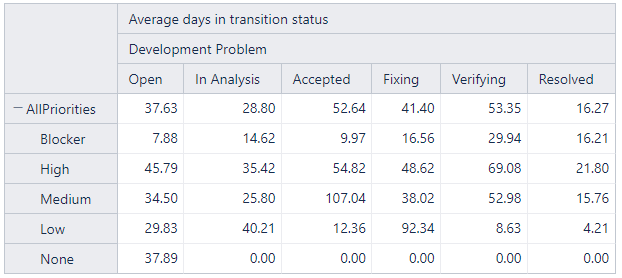# Table Cell Formatting per row and cell

Hello!
I hope you can help me with a case of cell formatting. I have created the table below and I want to apply a different cell formatting to each cell, depending on the status and the priority. For instance, an OPEN issue with priority BLOCKER should not be more than 4 days in that status and I want that cell highlighted in red, but if the priority is HIGH the threshold value is 8 days, so it also must be highlighted in red if the value in the cell exceeds it. The same goes for every combination of status and priority.So, is this possible?

I am using eazyBI version 6.4.1.

Thank you very much in advance!

hello @JReno

Not so long time ago I created a report similar to the one you are showing
It displays Overdue / Open / OK based on issue’s prio and number of days in a status.

Formula for “qwe” is (format: integer):

``````IIf(
IsEmpty([Measures].[Transition from status last date]),
DateDiffWorkdays(
[Measures].[Transition to status first date],
Now()
)
,
DateDiffWorkdays(
[Measures].[Transition to status first date],
[Measures].[Transition from status last date]
)
)
``````

Formula for “is it right” (format: default):

``````CASE
WHEN ([Priority].CurrentHierarchyMember.Name = "Lowest"
OR [Priority].CurrentHierarchyMember.Name = "Low" )
THEN
IIf(
[Measures].[qwe 44] > 15,
"Overdue",
IIF(
IsEmpty([Measures].[qwe 44]),
"",
IIf(
DateDiffWorkdays([Measures].[Transition to status first date],  Now()) <= 15
,
"Open",
"OK")
)
)
WHEN ([Priority].CurrentHierarchyMember.Name = "Medium")
THEN
IIf(
[Measures].[qwe 44] > 7,
"Overdue",
IIF(
IsEmpty([Measures].[qwe 44]),
"",
IIf(
DateDiffWorkdays([Measures].[Transition to status first date],  Now()) <= 7
,
"Open",
"OK")
)
)

ELSE
IIf(
[Measures].[qwe 44] > 3,
"Overdue",
IIF(
IsEmpty([Measures].[qwe 44]),
"",
IIf(
DateDiffWorkdays([Measures].[Transition to status first date],  Now()) <= 3
,
"Open",
"OK")
)
)
END
``````

Then you can format the cells:

Column “qwe” can be removed, it will not affect the “is it right”.

Hope it helps!Kind regards,
Vasile S.

Hello @VasileS,